# Test: Properties of Tangents

## 15 Questions MCQ Test Online MCQ Tests for Class 10 | Test: Properties of Tangents

Description
Attempt Test: Properties of Tangents | 15 questions in 15 minutes | Mock test for Class 10 preparation | Free important questions MCQ to study Online MCQ Tests for Class 10 for Class 10 Exam | Download free PDF with solutions
QUESTION: 1

### PT is a tangent from an external point P and PAB is a secant. If PT = 6cm and PA = 3cm, then the length of PB is __​

Solution:

We know that:
PT2 = PA X PB
therfore PB = PT2/PA = 36/3 = 12

QUESTION: 2

Solution:
QUESTION: 3

### In the given figure, AR = 5cm, BR = 4cm and AC = 11cm. What is the length of BC?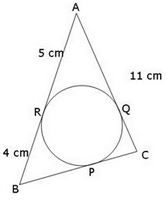​

Solution:

Since the sides of the triangles are tangent to the circle. BR = BP,AR = AQ and CP = CQ
So, BP = 4cm and AQ = 5
CQ = 11-5 = 6 = CP
BC = BP + CP = 4 + 6 = 10 cm

QUESTION: 4

In given figure, the length of BC is​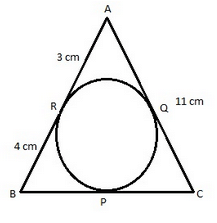Solution:
QUESTION: 5

Segment joining the points of contact of two parallel tangents

Solution:
QUESTION: 6

In a circle with centre O, AB and CD are two diameters perpendicular to each other. The length of chord AC is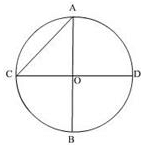Solution:
QUESTION: 7

If in the given figure radius of smaller and larger circles be 4 and 5 cm. Find the length of chord AB.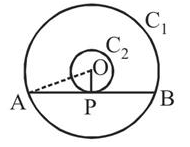Solution:
QUESTION: 8

A tangent PQ at a point P of a circle of radius 5 cm meets a line through the centre O at a point Q so that OQ = 12 cm. Length PQ is :

Solution:
QUESTION: 9

The maximum number of common tangents that can be drawn to two circles intersecting at two distinct point is

Solution:
QUESTION: 10

In a circle of radius 7cm, tangent PT is drawn from point P such that PT = 24cm. If O is the centre of the circle, then the length of OP is:​

Solution:
QUESTION: 11

In the figure, PQ is a tangent at a point R of the circle with centre O. If ∠TRQ = 30°.The measure of ∠PRS is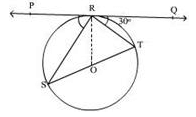Solution:
QUESTION: 12

In the given figure, length of BC is: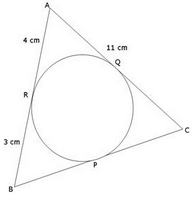Solution:
QUESTION: 13

ABC is a right triangle right angled at B such that BC = 6 cm and AB = 8 cm. The radius of its incircle is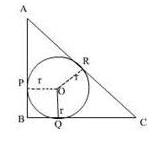Solution:

By pythagoras theorem ,
H2 = P2 + B2
=36 + 64=100
H=10
We have a square BPOQ so all sides are equal.
Since tangents from a point are equal in length
Let AP = AR = x
BP=BQ=y
RC = QC = z
x + y = 8
x = 8 - y
x + z = 10 ⇒ 8 - y + z = 10 ⇒ z = 2 + y
y + z = 6 ⇒ y + 2 + y = 6 ⇒ y = 2
x = 6, z= 4
Since BP = OQ = y = 2

QUESTION: 14

Quadrilateral ABCD circumscribes a circle as shown in figure. The side of the quadrilateral which is equal to AP + BR is: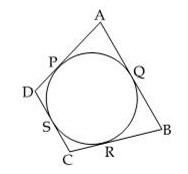Solution:
QUESTION: 15

If the angle between two radii of a circle is 100°, then angle between the tangents at the ends of those radii is:​

Solution:Use Code STAYHOME200 and get INR 200 additional OFF Use Coupon Code